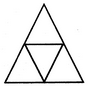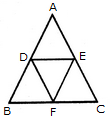# Non Verbal Reasoning - Analytical Reasoning - Discussion

Discussion Forum : Analytical Reasoning - Section 1 (Q.No. 29)
29.

Find the number of triangles in the given figure.4
5
6
7
Explanation:

The figure may be labelled as shown.The simplest triangles are ADE, BDF, DEF and EFC i.e. 4 in number.

There is only one triangle ABC composed of four components.

Thus, there are 4+1 = 5 triangles in the given figure.

Discussion:
Be the first person to comment on this question !